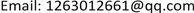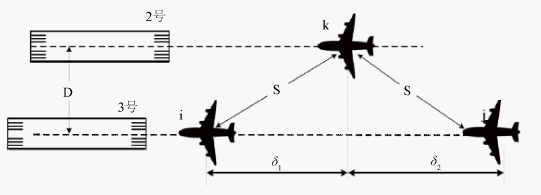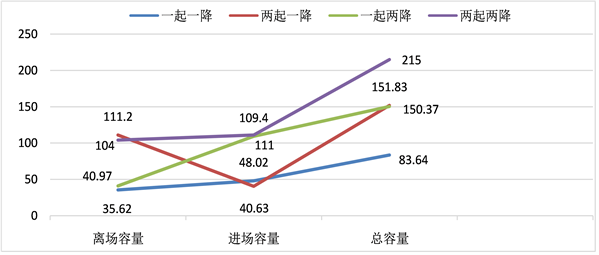﻿ 基于民航多种跑道运行模式下军航中距双跑道应用探究 Research on the Application of Middle Distance Dual Runway Based on Multiple Runway Operation Modes of Civil Aviation

Open Journal of Transportation Technologies
Vol. 09  No. 03 ( 2020 ), Article ID: 35710 , 9 pages
10.12677/OJTT.2020.93025

Research on the Application of Middle Distance Dual Runway Based on Multiple Runway Operation Modes of Civil Aviation

Xianghui Geng, Di Shen, Tianwei Ren

Air Force Engineering University, Xi’an ShaanxiReceived: May 4th, 2020; accepted: May 15th, 2020; published: May 22nd, 2020ABSTRACT

The establishment of dual runways and their safe and efficient operation in the modern military environment is one of the important ways to improve the capacity of military airports and their anti-damage capability in wartime, and to meet the needs of multiple models, high strength and large flight flow. As the construction and operation of the military middle distance dual runway airport is in the initial stage of development, the infrastructure and operation mechanism are not perfect. In order to better promote the rapid generation of combat effectiveness of the middle distance dual runway airport and explore a development path of the middle distance dual runway that is in line with the actual combat situation, this paper evaluates the runway capacity by using mathematical models based on the operation examples of the middle distance dual runway under different modes of civil aviation, comparing and combining with the actual situation of the military airport. On the basis of comparative analysis of runway capacity under multiple operation modes, the implementation suggestions for future military middle distance dual runway operation are put forward, which provides some reference for its development and application.

Keywords:Military Airports, Dual Runway, Operating Mode1. 前言

2. 平行双跑道运行模式

2.1. 民航双跑道运行模式的确定

2.2. 军航双跑道运行模式的确定

3. 中距跑道容量分析

3.1. 一起一降模式

${T}_{ij}\left(AI\right)=\mathrm{max}\left({}_{a}T{}_{take},{}_{a}T{}_{\mathrm{min}}\right)$ (1)

$E\left[T\left(AI\right)\right]=\underset{i=1}{\overset{n}{\sum }}\underset{j=1}{\overset{n}{\sum }}{p}_{ij}{T}_{ij}\left(AI\right)$ (2)

${C}_{AI}=\frac{1}{E\left[T\left(AI\right)\right]}$ (3)

${T}_{ij}\left(DE\right)=\mathrm{max}\left({}_{d}T{}_{take},{}_{d}T{}_{\mathrm{min}}\right)$ (4)

$E\left[T\left(DE\right)\right]=\underset{i=1}{\overset{n}{\sum }}\underset{j=1}{\overset{n}{\sum }}{p}_{ij}{T}_{ij}\left(DE\right)$ (5)

${C}_{DE}=\frac{1}{E\left[T\left(DE\right)\right]}$ (6)

${C}_{YQYJ}={C}_{DE}+{C}_{AI}$ (7)

3.2. 两起一降模式

${C}_{HH}={C}_{AI}\left(1+\underset{i=1}{\overset{n}{\sum }}\underset{j=1}{\overset{n}{\sum }}{p}_{ij}{\theta }_{ij\mathrm{max}}\right)$ (8)

${C}_{LQYJ}={C}_{DE}+{C}_{HH}$ (9)

3.3. 两降一起模式Figure 1. Related parallel approach mode

${T}_{ij}\left(AI\right)=\mathrm{max}\left\{\begin{array}{l}{}_{a}T{}_{follow}\\ {}_{a}T{}_{take}\\ {}_{a}T{}_{ig}+{}_{a}T{}_{gj}\end{array}$ (10)

$T\left(AI\right)=E\left[{T}_{ij}\left(AI\right)\right]=\underset{i=1}{\overset{n}{\sum }}\underset{j=1}{\overset{n}{\sum }}\underset{g=1}{\overset{n}{\sum }}{p}_{ij}{p}_{g}{T}_{ij}\left(AI\right)$ (11)

$C\left(AI\right)=\frac{1}{T\left(AI\right)}$ (12)

${\theta }_{ij\mathrm{max}}$ 表示同一条跑道上的一对降落飞机间可以插入的最大起飞飞机数，则当一条跑道用于起飞/降落混合操作，另一条仅用于降落时的容量为：

${C}_{YQLJ}=C\left(AI\right)\left(2+\underset{i=1}{\overset{n}{\sum }}\underset{j=1}{\overset{n}{\sum }}{p}_{ij}{\theta }_{ij\mathrm{max}}\right)$ (13)

3.4. 两起两降模式

${C}_{LQLJ}=2\left[C\left(AI\right)\left(1+\underset{i=1}{\overset{n}{\sum }}\underset{j=1}{\overset{n}{\sum }}{p}_{ij}{\theta }_{ij\mathrm{max}}\right)\right]$ (14)

4. 实例分析

4.1. 容量分析

4.1.1. 一起一降Table 1. Regulations on minimum takeoff safety interval of aircraft (unit: s)Table 2. Maximum number of aircrafts inserted in aircraft safety interval

$E\left[{T}_{ij}\left(DE\right)\right]=101.08\text{\hspace{0.17em}}\text{s}/架$

4.1.2. 两起一降

${C}_{HH}=116.2架/\text{h}$

${C}_{LQYJ}=151.83架/\text{h}$

4.1.3. 一起两降Table 3. Regulations on the approach aircraft interval of mixed operation runway

$C\left(AI\right)=42.84架/\text{h}$

${C}_{YQLJ}=C\left(AI\right)+C\left(HH\right)=C\left(AI\right)+C\left(AI\right)\left(1+\underset{i＝1}{\overset{n}{\sum }}\underset{j＝1}{\overset{n}{\sum }}{p}_{ij}{\theta }_{ij\mathrm{max}}\right)$

${C}_{YQLJ}=150.37架/\text{h}$

4.1.4. 两起两降

${C}_{LQLJ}=215架/\text{h}$

4.2. 误差分析

① 未考虑到天气因素，在特殊复杂天气情况下，军航机场除特殊科目训练和重要任务外，减少或者不进行飞行，从而使得机场运行容量降低。

② 军航实际运行过程中，为避免非战斗损失和降低事故率，不同管制员会根据自身能力和经验不同，适当增加实际运行下的安全间隔，使得航空器之间不那么紧凑，提高跑道运行安全性，但使得对单架航空器的服务时间增多，减少了机场运行容量。

③ 插空放飞飞机时实际可行性，最大理论容量评估过程中，对可插空飞行的空档插入最大可飞行数量，实际操作过程中，并没有无限等待起飞的航空器，因而插空放飞飞机数量的不足，直接导致了跑道离场容量的不足。

4.3. 不同模式下跑道容量对比Figure 2. Runway capacity in different modes

5. 军航应用设想

(1) 作战开始阶段，选择两起一降和两起两降的双跑道运行模式。对于军航任务的突然性以及对出动时间的较高要求，即务必在第一时间起飞，最快到达目的地域实施作战行动，而期间对着陆的要求不高。因而，在任务开始阶段，可以选择两起一降和两起两降的双跑道运行模式。此时，既能满足作战飞机迅速起飞的战场需求，又能在一定程度上给少量来场航空器留有着陆空间。

(2) 作战结束阶段或回场补给阶段，可以选择一起两降和两起两降的双跑道运行模式。在任务结束后回场归队或者任务执行过程中飞机来场加油补给，特别是飞机大规模来场，在这一阶段，飞机可能会在空战中受创，油量不足，在运输过程中遇到突发状况而必须尽快着陆等诸多情况，此时，对机场的着陆容量提出的较高要求。因此，此时可以选择一起两降和两起两降的双跑道运行模式。

(3) 在平时训练过程中，可以采取一起一降的双跑道运行模式。由于军航的飞机数量并不像民航那么多，并不需要过大的跑道容量且双跑道混合运行对于机场导航设备精度，管制员指挥能力，飞行员技术水平提出了较高的要求，因而在平常的训练过程中，可以采取一起一降的双跑道运行模式，既能保证比平常单跑道更大的运行容量，另一条跑道随时可以应对突发情况，可以插空加入飞机来起飞或者降落，又能保证日常训练的安全性。

6. 结束语

Research on the Application of Middle Distance Dual Runway Based on Multiple Runway Operation Modes of Civil Aviation[J]. 交通技术, 2020, 09(03): 207-215. https://doi.org/10.12677/OJTT.2020.93025

1. 1. 蒋兵, 胡明华, 田勇, 等. 机场跑道容量评估模型和估计方法的进一步研究[J]. 交通运输工程学报, 2003, 3(2): 80-83.

2. 2. 陈勇. 空域容量评估模型和优化方法研究[D]: [博士学位论文]. 北京: 北京航空航天大学, 2005.

3. 3. 徐肖豪, 于跃, 黄宝军, 等. 不同运行模式的近距平行跑道容量分析[J]. 中国民航大学学报, 2012, 30(6): 34 -39.

4. 4. 孟祥伟, 韩宝华, 王宏远, 等. 近距平行跑道最大容量模型[J]. 空军工程大学学报(自然科学版), 2016, 17(4): 7-12.

5. 5. 戴喆, 余敏建, 甘旭升, 军用机场平行双跑道应用需求及运行模式[J]. 火力与指挥控制, 2017, 42(12): 50-54.

6. 6. 李捷, 苟景全. 信息化条件下双跑道机场基地化保障研究[J]. 空军军事学术, 2013, 54(4): 93-95.

7. 7. Li, L., Li, F. and Chen, X.Q. (2017) Study on the Configuration and Capacity of the Lateral Runway Based on the Airport Green Operation. Iop Conference Series: Earth and Environmental Science, 63, 12-32. https://doi.org/10.1088/1755-1315/63/1/012032

8. 8. 孔航, 胡明华, 姚逸, 等, 不同运行模式下跑道容量评估技术研究[J]. 航空计算技术, 2017, 47(4): 57-61.

9. 9. 孙海勇, 刘裕旭. 不同运行模式下的远距跑道容量研究[J]. 航空计算技术, 2019, 49(5): 60-66.

10. 10. 陈志杰. 空域管理理论与方法[M]. 北京: 科技出版社, 2012.